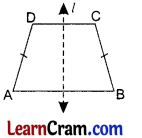# DAV Class 7 Maths Chapter 13 Worksheet 3 Solutions

The DAV Class 7 Maths Book Solutions and DAV Class 7 Maths Chapter 13 Worksheet 3 Solutions of Symmetry offer comprehensive answers to textbook questions.

## DAV Class 7 Maths Ch 13 WS 3 Solutions

Question 1.
How many lines of symmetry will a square have? Explain by paper folding.Take a square paper sheet and fold it 4 times along the dotted lines l1 l2, l3 and l4. Hence, the square has 4 lines of symmetry.

Question 2.
Give reasons to show how many lines of symmetry will a square have.
A square has 4 lines of symmetry as it has 4 equal sides and 4 equal lines.

Question 3.
How many lines of symmetry will a general quadrilateral have?
General quadrilateral has no line of symmetry.Question 4.
How many lines of symmetry will a kite have? Draw the lines of symmetry of a kite.